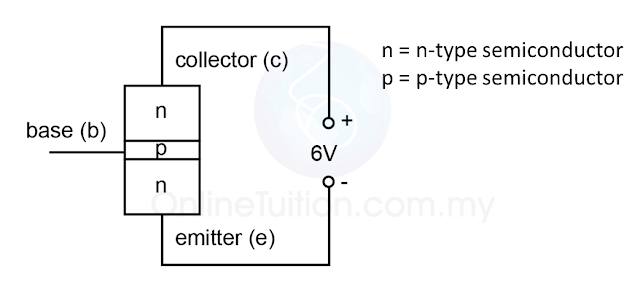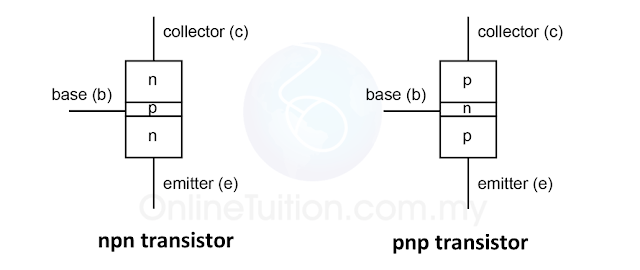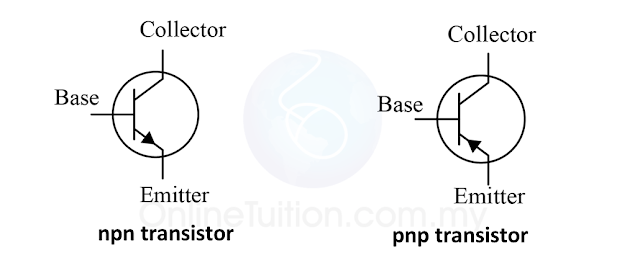# Transistors

### Transistors

1. A transistor is a double p-n junction semiconductor with three terminals,
1. the emitter (e),
2. the base (b)
3. the collector (c).
2. Figure 1 below shows the illustration of a transistor. It looks like a combination of 2 p-n junction diodes.(Figure 1)
1. In a transistor, the emitter emits charge carriers (free electrons or holes).
2. The charge carriers move towards the base.
3. Under certain condition, a large amount of the charge carriers will pass through the thin base layer and be collected by the collector.

### Types of the Transistors

1. There are 2 types of transistors:
1. npn transistor
2. pnp transistor
2. Figure 2 below shows the illustration of the npn and pnp transistor and Figure 3 below shows the symbol of both npn and pnp transistor.
3. For the symbol of the transistor, the arrow shows the direction of the current. Take note that, for the emitter and base, the current always flows from the positive terminal to the negative terminal.(Figure 2: Illustration of the npn and pnp transistor)(Figure 3: Symbol of the npn and pnp transistor)

### How a Transistor Work?(Figure 4)
1. In Figure 4 above, there are 2 circuits in the connection:
1. the base circuit
2. the collector circuit
2. The base circuit is forward bias whereas the collector circuit is reverse bias (This will be discussed in “The Connection of a Transistor”).
3. The table below shows the response of bulb 1 (B1) and bulb 2 (B2) when switch 1 (S1) and switch 2 (S2) are closed.
 S1 S2 B1 B2 Open Open Does not light up Does not light up Close Open Light up Does not light up Open Close Does not light up Does not light up Close Close Light up Light up
1. From the table, we can see that the collector circuit is controlled by the base circuit.
2. Current will flow in the collector circuit only when the base circuit is closed.